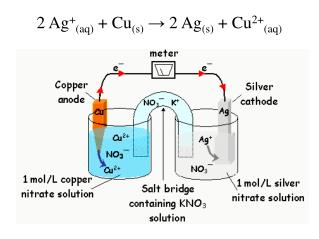DownloadDownload Presentation2 Ag + (aq) + Cu (s) → 2 Ag (s) + Cu 2+ (aq)

# 2 Ag + (aq) + Cu (s) → 2 Ag (s) + Cu 2+ (aq)

Télécharger la présentation## 2 Ag + (aq) + Cu (s) → 2 Ag (s) + Cu 2+ (aq)

- - - - - - - - - - - - - - - - - - - - - - - - - - - E N D - - - - - - - - - - - - - - - - - - - - - - - - - - -
##### Presentation Transcript

1. 2 Ag+(aq) + Cu(s) → 2 Ag(s) + Cu2+(aq)

2. Reduction Potential and Cells

3. Explore the Standard Reduction Potential Chart • Predict spontaneous using Reduction Potential.

4. Spontaneous rxns occurs without added energy. 2 Ag+(aq) + Cu(s) → Ag(s) + Cu2+(aq) → 2 Ag(s) + Cu2+(aq) no reaction Ag+ions can oxidizeCu metal. Cu2+cannot oxidize Ag metal.

5. Electrochemical cells are described in terms of their voltage. (formerly called Emf – electromotive force). Voltage (Eocell) found using Reduction Potentials. Potentials (Eo) for eachsubstance are calculated by competing with a hydrogen standard in a cell. 2H+(aq) + 2e– ↔ H2(g)

6. Reduction Potential Chart Order of ability to react with other compounds.

7. (+) Eo - greater tendency to accept electrons vs. H+. X – reduced Hydrogen – oxidized (-) Eo - lesser tendency to accept electrons. X – oxidized Hydrogen – reduced Greater positive number is reduced. Eo = +0.34 V H2(g) / H+(aq) // Cu2+(aq) / Cu(s) Zn(s) / Zn2+(aq) // H+(aq) / H2(g) Eo = -0.76 V

8. E°cell- sum of potentials of each half-cell. E°cell = E°ox + E°red Note: table lists reduction potentials. Oxidationpotentials are the reverse – switch sign. (+) E°cell– spontaneous reaction. (-) E°cell– non – spontaneous reaction.

9. What is the cell potential for a silver-copper cell? Ag+(aq) + 1e– → Ag(s) E° = +0.80 V red → Cu(s) – Cu2+(aq) + 2e– E° = 0.34 V ox + E°cell = E°ox + E°red = - 0.34 + 0.80 + 0.46

10. A cell of zinc and gold metal as electrodes: a) What is the cathode and what is the anode?b) What is the net reaction?c) What is the line notation for the cell?d) What is the cell potential?

11. [ ] ×2 Au3+(aq) + 3e– → Au(s) E° = +1.50 V red [ ] ×3   → Zn(s) Zn2+(aq) + 2e– - E° = 0.76 V ox + Zn(s) / Zn2+(aq) // Au3+(aq) / Au(s) 2 Au3+(aq) + 3 Zn(s) → 2 Au(s) + 3 Zn2+(aq) We do NOT multiply the voltage. + 2.26 E°cell = E°ox + E°red = + 0.76 + 1.50

12. 2 Ag+(aq) + Cu(s) → Ag(s) + Cu2+(aq) → 2 Ag(s) + Cu2+(aq) E°c = +0.46 no reaction E°c = - 0.46

13. Will tin strips in hydrochloric acid react? Sn(s) + H+(aq) → ?? 2 Sn(s) + H+(aq) → Sn2+(aq) + H2(g)

14. Given the following experimental data, arrange the following in increasing order of oxidizing ability. Co2+ 1. + In(s) → Co(s) + In2+ 2. + Co(s) → Cu(s) + Co2+ 3. Cu2+ + → no reaction Cu2+ Pd(s) Weak Oxidizer In2+ + 2e– → In(s) Co2+ + 2e– → Co(s) Cu2+ + 2e– → Cu(s) Strong Oxidizer Pd2+ + 2e– → Pd(s) Remember the strong oxidizer is reduced